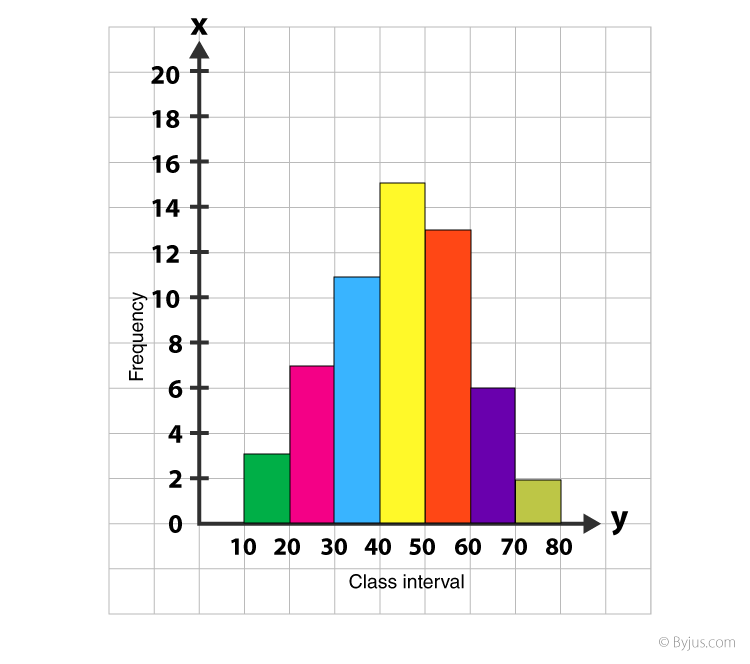# Sandeep Garg Solutions Class 11 Economics Chapter 4 - Graphic Presentation

Sandeep Garg Class 11 Economics Solutions Chapter 4 – Graphic Presentation is illustrated by the professional Economics educator from the contemporary edition of Sandeep Garg Economics Class 11 textbook solutions.

We at BYJU’S provide Sandeep Garg Economics Class 11 Solutions to give a comprehensive insight into the subject to the students. These insights will be a valuable advantage to students while completing their homework or while studying for their exams.

In this article, we are providing you with the solution from the tabular presentation which will be helpful for the students to score well in the board exams.

## Sandeep Garg Solutions Class 11 – Chapter 4

Question 1

Prepare a line frequency graph from the following data.

 Marks 20 30 40 50 60 70 80 Frequencies 7 9 11 12 14 15 17

The given data can be presented in a line frequency graph as follows: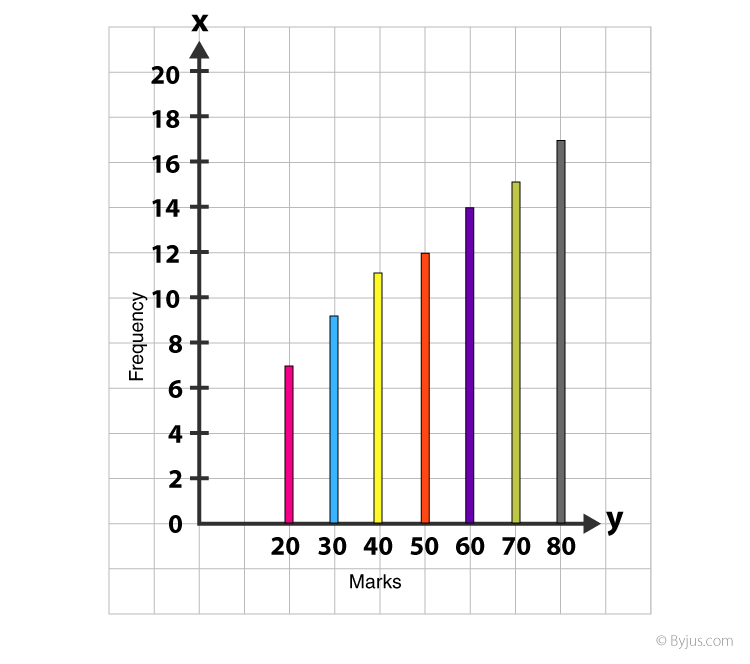Question 2

Represent a histogram from the following data.

 Marks 0−10 10−20 20−30 30−40 40−50 No. of Students 15 30 58 45 35

The given data can be represented with the help of a histogram as follows: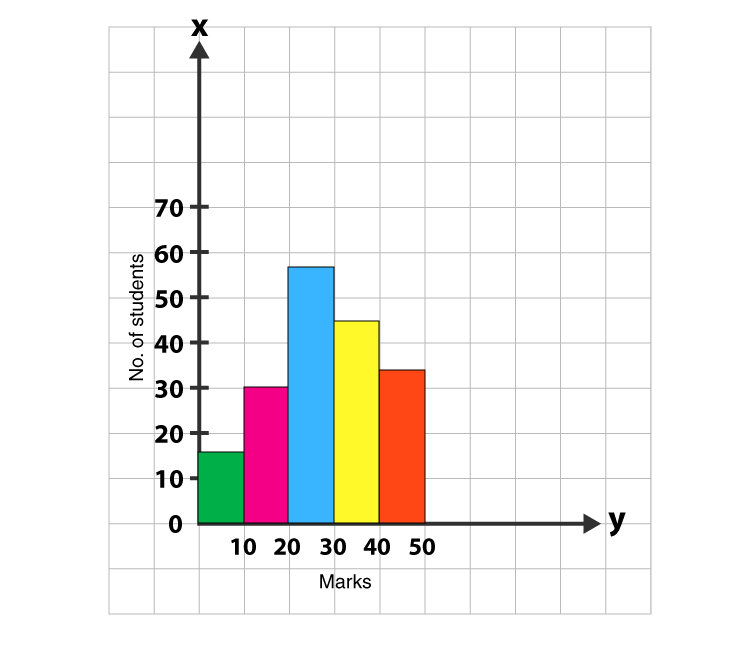Question 3

Make a histogram from the following frequency.

 Class Intervals 2.5 7.5 12.5 17.5 22.5 Frequencies 5 10 30 15 6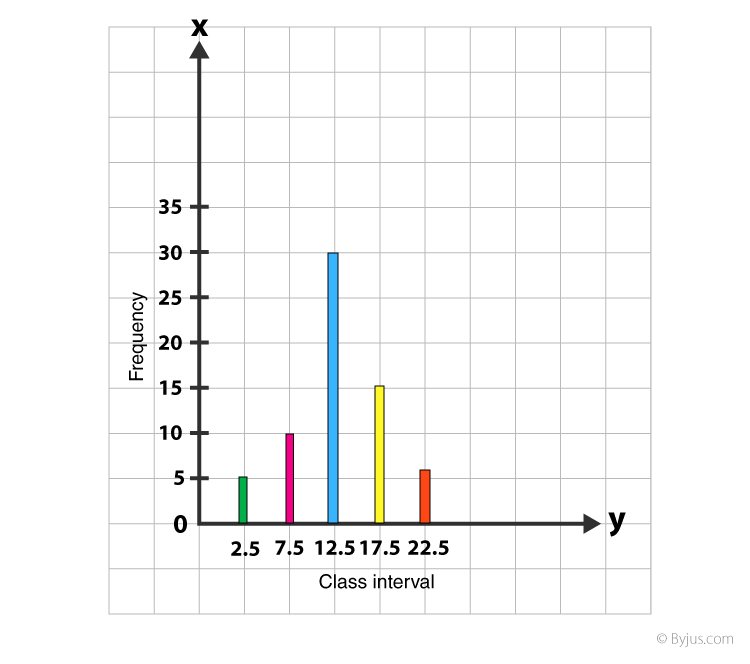Here, the gap between the two mid-values is 5. So, it’s half difference (2.5) will be subtracted and added from each mid-point to get the below-mentioned class intervals.

 Mid-points Class intervals Frequencies 2.5 7.5 12.5 17.5 22.5 0 − 5 5 − 10 10 − 15 15 − 20 20 − 25 5 10 30 15 6

Question 4

Prepare a polygon with the help of the following frequencies.

 Mid-Values 5 15 25 35 45 55 65 75 Frequency 4 10 16 22 25 12 7 2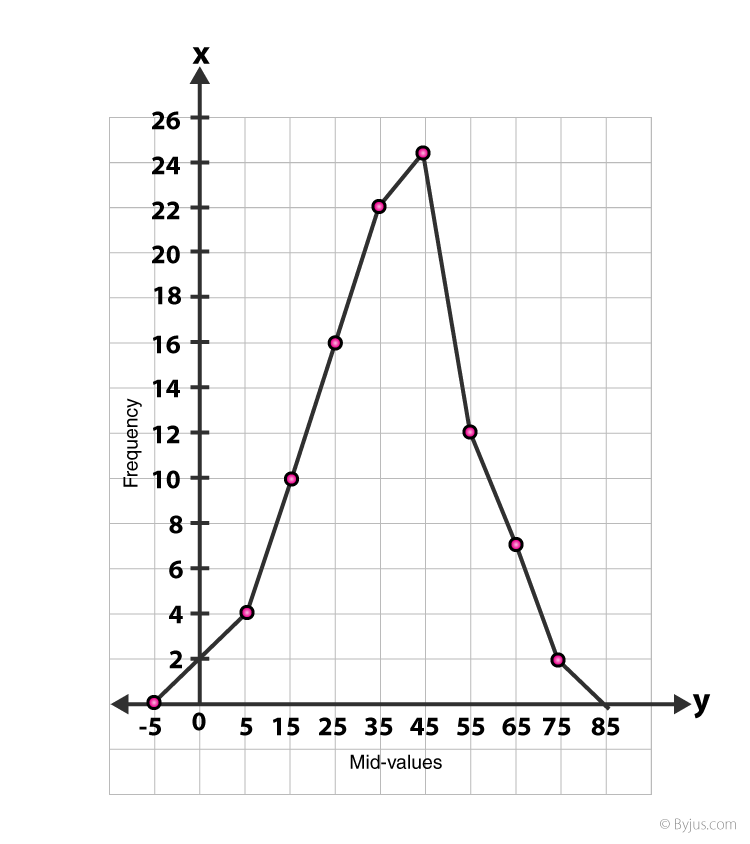Question 5

Arrange a frequency curve of the following details:

 Class-interval 10−20 20−30 30−40 40−50 50−60 60−70 70−80 Frequencies 3 7 11 15 13 6 2